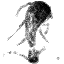Repository for Ideas & Research
Open Source GIS, Hydrologic Modeling, Optimization

# How to fill no data pixels in a raster map in GRASS GIS

I need to fill onshore NULL (no data) pixels in a DEM raster map shown below.The first step is to fill no data pixels by interpolation.

``````g.region rast=dem1km
r.fillnulls input=dem1km output=dem1km2 method=bilinear``````

However, r.fillnulls fills not only small islands of NULL pixels, but also any NULL pixels outside the valid raster boundaries. The output from r.fillnulls will be rectangular regardless of the original shape of the input raster map.I need to create a mask that only covers the original data extent, but it should also include no data areas. First, create a mask using the original DEM raster map.

``r.mapcalc expression='mask1km=if(!isnull(dem1km),1,null())'``Convert this raster mask to vector.

``r.to.vect input=mask1km output=mask1km type=area``There are island areas where no data pixels are. These areas are not assigned a centroid, so let’s assign a centroid to them.

``v.centroids input=mask1km output=mask1km2``Create a new column “dissolve” and assign the same value of 1 to this column for all areas. Then, dissolve all areas by this column.

``````v.db.addcolumn map=mask1km2 column='dissolve int'Convert the dissolved vector to raster.

``v.to.rast input=mask1km3 output=mask1km3 use=val``Extract the filled DEM by the final mask.

``r.mapcalc expression='dem1km3=if(!isnull(mask1km3),dem1km2,null())'``